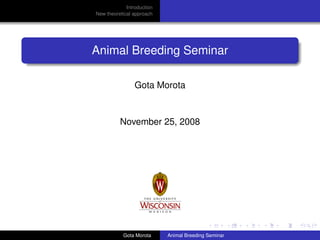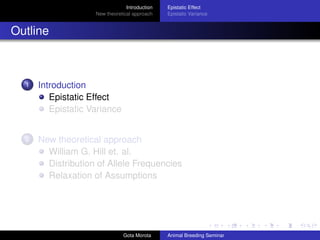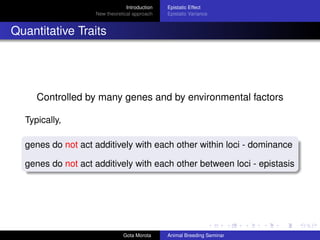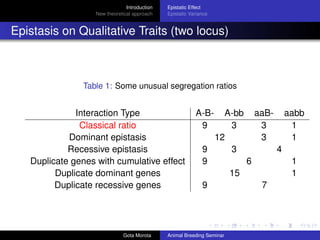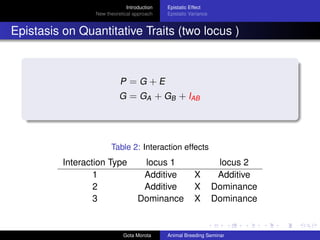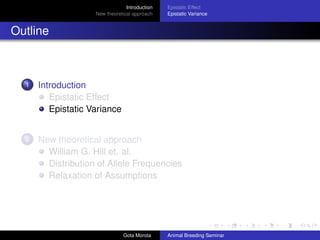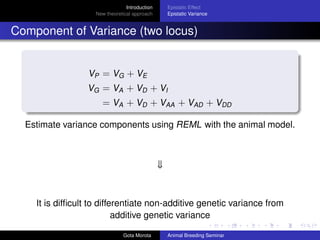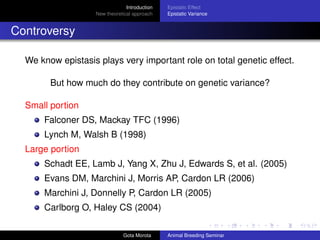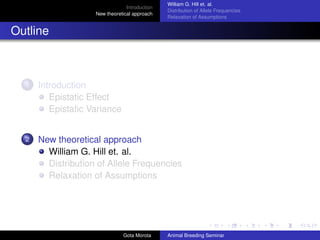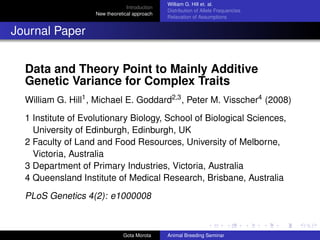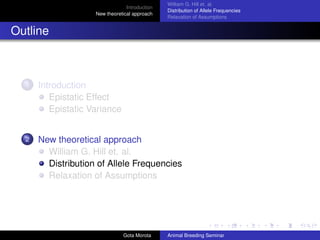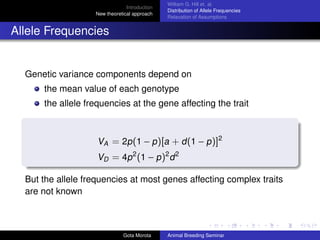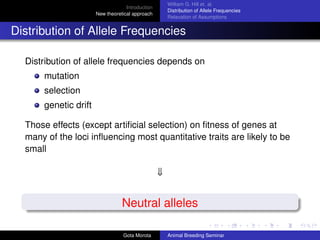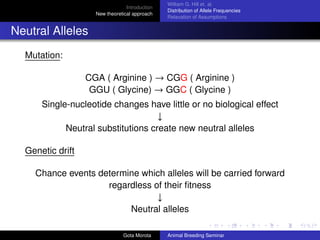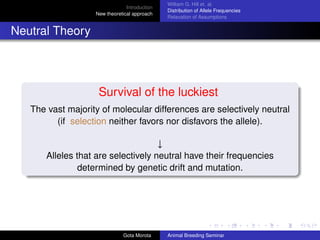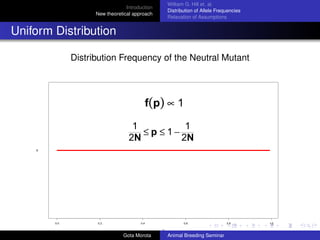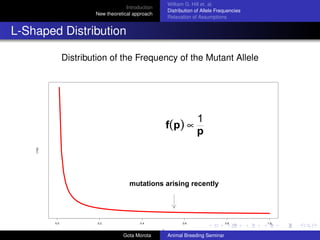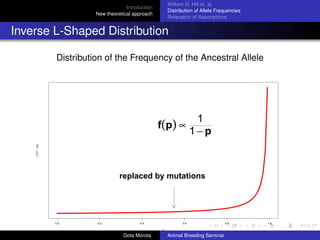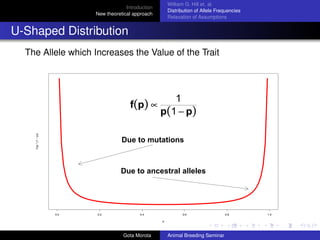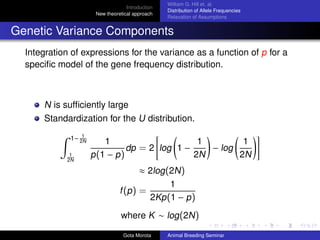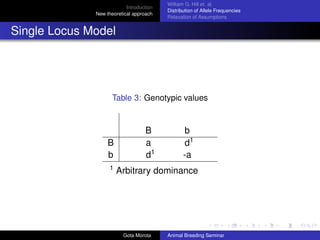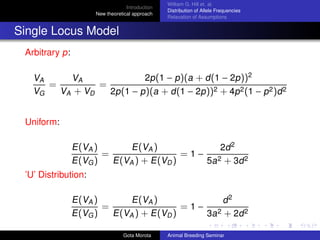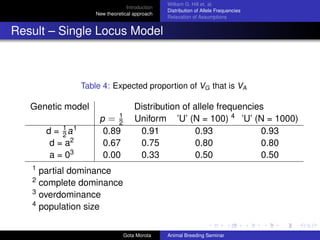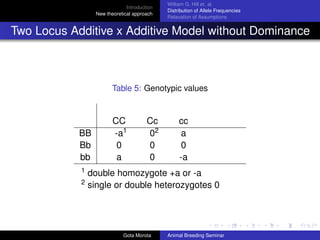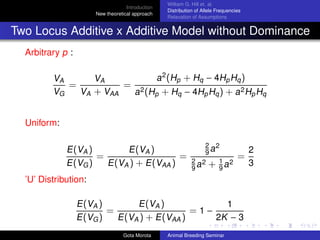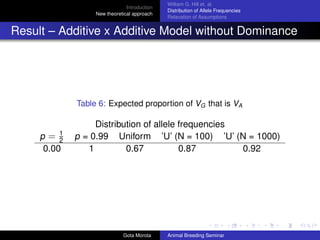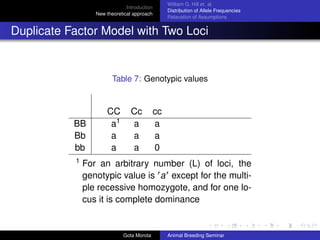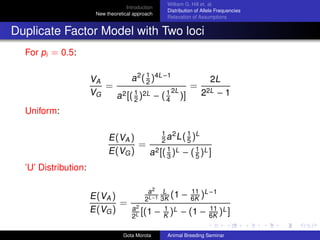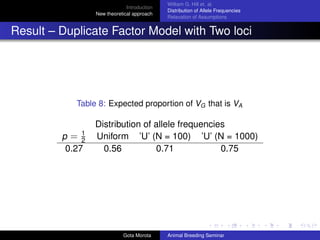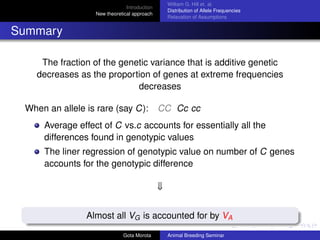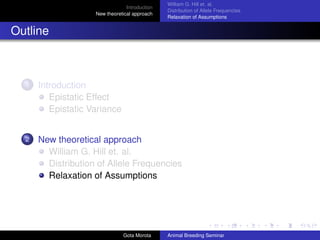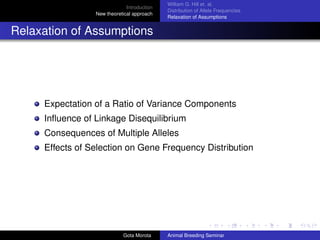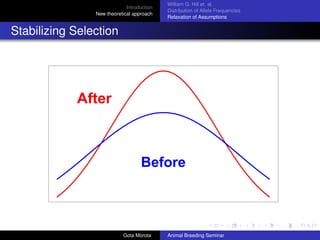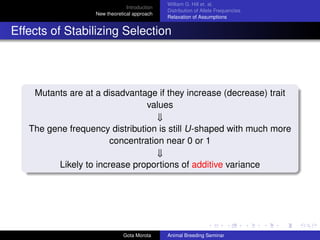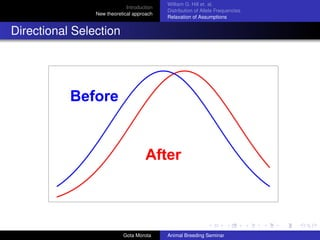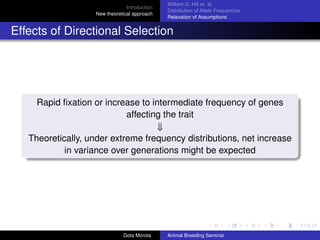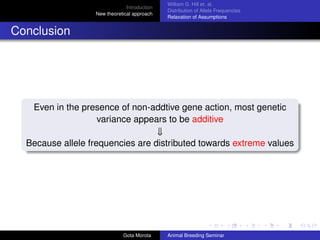1 of 38

### Hill, W. G, et al. 2008. Data and Theory Point to Mainly Additive Genetic Variance for Complex Traits. PLoS Genetics 4(2): e1000008.

1. Introduction New theoretical approach Animal Breeding Seminar Gota Morota November 25, 2008 Gota Morota Animal Breeding Seminar
2. Introduction New theoretical approach Epistatic Effect Epistatic Variance Outline 1 Introduction Epistatic Effect Epistatic Variance 2 New theoretical approach William G. Hill et. al. Distribution of Allele Frequencies Relaxation of Assumptions Gota Morota Animal Breeding Seminar
3. Introduction New theoretical approach Epistatic Effect Epistatic Variance Quantitative Traits Controlled by many genes and by environmental factors Typically, genes do not act additively with each other within loci - dominance genes do not act additively with each other between loci - epistasis Gota Morota Animal Breeding Seminar
4. Introduction New theoretical approach Epistatic Effect Epistatic Variance Epistasis on Qualitative Traits (two locus) Table 1: Some unusual segregation ratios Interaction Type Classical ratio Dominant epistasis Recessive epistasis Duplicate genes with cumulative effect Duplicate dominant genes Duplicate recessive genes Gota Morota A-B9 A-bb aaB- aabb 3 3 1 12 3 1 9 3 4 9 6 1 15 1 9 7 Animal Breeding Seminar
5. Introduction New theoretical approach Epistatic Effect Epistatic Variance Epistasis on Quantitative Traits (two locus ) P =G+E G = GA + GB + IAB Table 2: Interaction effects Interaction Type 1 2 3 locus 1 Additive Additive Dominance Gota Morota X X X locus 2 Additive Dominance Dominance Animal Breeding Seminar
6. Introduction New theoretical approach Epistatic Effect Epistatic Variance Outline 1 Introduction Epistatic Effect Epistatic Variance 2 New theoretical approach William G. Hill et. al. Distribution of Allele Frequencies Relaxation of Assumptions Gota Morota Animal Breeding Seminar
7. Introduction New theoretical approach Epistatic Effect Epistatic Variance Component of Variance (two locus) VP = VG + VE VG = VA + VD + VI = VA + VD + VAA + VAD + VDD Estimate variance components using REML with the animal model. ⇓ It is difﬁcult to differentiate non-additive genetic variance from additive genetic variance Gota Morota Animal Breeding Seminar
8. Introduction New theoretical approach Epistatic Effect Epistatic Variance Controversy We know epistasis plays very important role on total genetic effect. But how much do they contribute on genetic variance? Small portion Falconer DS, Mackay TFC (1996) Lynch M, Walsh B (1998) Large portion Schadt EE, Lamb J, Yang X, Zhu J, Edwards S, et al. (2005) Evans DM, Marchini J, Morris AP, Cardon LR (2006) Marchini J, Donnelly P, Cardon LR (2005) Carlborg O, Haley CS (2004) Gota Morota Animal Breeding Seminar
9. Introduction New theoretical approach William G. Hill et. al. Distribution of Allele Frequencies Relaxation of Assumptions Outline 1 Introduction Epistatic Effect Epistatic Variance 2 New theoretical approach William G. Hill et. al. Distribution of Allele Frequencies Relaxation of Assumptions Gota Morota Animal Breeding Seminar
10. Introduction New theoretical approach William G. Hill et. al. Distribution of Allele Frequencies Relaxation of Assumptions Journal Paper Data and Theory Point to Mainly Additive Genetic Variance for Complex Traits William G. Hill1 , Michael E. Goddard2,3 , Peter M. Visscher4 (2008) 1 Institute of Evolutionary Biology, School of Biological Sciences, University of Edinburgh, Edinburgh, UK 2 Faculty of Land and Food Resources, University of Melborne, Victoria, Australia 3 Department of Primary Industries, Victoria, Australia 4 Queensland Institute of Medical Research, Brisbane, Australia PLoS Genetics 4(2): e1000008 Gota Morota Animal Breeding Seminar
11. Introduction New theoretical approach William G. Hill et. al. Distribution of Allele Frequencies Relaxation of Assumptions Outline 1 Introduction Epistatic Effect Epistatic Variance 2 New theoretical approach William G. Hill et. al. Distribution of Allele Frequencies Relaxation of Assumptions Gota Morota Animal Breeding Seminar
12. Introduction New theoretical approach William G. Hill et. al. Distribution of Allele Frequencies Relaxation of Assumptions Allele Frequencies Genetic variance components depend on the mean value of each genotype the allele frequencies at the gene affecting the trait VA = 2p (1 − p )[a + d (1 − p )]2 VD = 4p 2 (1 − p )2 d 2 But the allele frequencies at most genes affecting complex traits are not known Gota Morota Animal Breeding Seminar
13. William G. Hill et. al. Distribution of Allele Frequencies Relaxation of Assumptions Introduction New theoretical approach Distribution of Allele Frequencies Distribution of allele frequencies depends on mutation selection genetic drift Those effects (except artiﬁcial selection) on ﬁtness of genes at many of the loci inﬂuencing most quantitative traits are likely to be small ⇓ Neutral alleles Gota Morota Animal Breeding Seminar
14. William G. Hill et. al. Distribution of Allele Frequencies Relaxation of Assumptions Introduction New theoretical approach Neutral Alleles Mutation: CGA ( Arginine ) → CGG ( Arginine ) GGU ( Glycine) → GGC ( Glycine ) Single-nucleotide changes have little or no biological effect ↓ Neutral substitutions create new neutral alleles Genetic drift Chance events determine which alleles will be carried forward regardless of their ﬁtness ↓ Neutral alleles Gota Morota Animal Breeding Seminar
15. William G. Hill et. al. Distribution of Allele Frequencies Relaxation of Assumptions Introduction New theoretical approach Neutral Theory Survival of the luckiest The vast majority of molecular differences are selectively neutral (if selection neither favors nor disfavors the allele). ↓ Alleles that are selectively neutral have their frequencies determined by genetic drift and mutation. Gota Morota Animal Breeding Seminar
16. William G. Hill et. al. Distribution of Allele Frequencies Relaxation of Assumptions Introduction New theoretical approach Uniform Distribution Distribution Frequency of the Neutral Mutant f(p) ∝ 1 m 1 1 ≤p ≤1− 2N 2N 0.0 0.2 0.4 0.6 p Gota Morota Animal Breeding Seminar 0.8 1.0
17. William G. Hill et. al. Distribution of Allele Frequencies Relaxation of Assumptions Introduction New theoretical approach L-Shaped Distribution Distribution of the Frequency of the Mutant Allele 1 p (1/p) f(p) ∝ mutations arising recently 0.0 0.2 0.4 0.6 p Gota Morota Animal Breeding Seminar 0.8 1.0
18. William G. Hill et. al. Distribution of Allele Frequencies Relaxation of Assumptions Introduction New theoretical approach Inverse L-Shaped Distribution Distribution of the Frequency of the Ancestral Allele 1 1−p (1/(1 − p)) f(p) ∝ replaced by mutations 0.0 0.2 0.4 0.6 p Gota Morota Animal Breeding Seminar 0.8 1.0
19. William G. Hill et. al. Distribution of Allele Frequencies Relaxation of Assumptions Introduction New theoretical approach U-Shaped Distribution The Allele which Increases the Value of the Trait 1/(p * (1 − p)) f(p) ∝ 1 p(1 − p) Due to mutations Due to ancestral alleles 0.0 0.2 0.4 0.6 p Gota Morota Animal Breeding Seminar 0.8 1.0
20. Introduction New theoretical approach William G. Hill et. al. Distribution of Allele Frequencies Relaxation of Assumptions Genetic Variance Components Integration of expressions for the variance as a function of p for a speciﬁc model of the gene frequency distribution. N is sufﬁciently large Standardization for the U distribution. 1 1− 2N 1 2N 1 p (1 − p ) dp = 2 log 1 − 1 1 − log 2N 2N ≈ 2log (2N ) f (p ) = 1 2Kp (1 − p ) where K ∼ log (2N ) Gota Morota Animal Breeding Seminar
21. Introduction New theoretical approach William G. Hill et. al. Distribution of Allele Frequencies Relaxation of Assumptions Single Locus Model Table 3: Genotypic values B b 1 B a d1 b d1 -a Arbitrary dominance Gota Morota Animal Breeding Seminar
22. Introduction New theoretical approach William G. Hill et. al. Distribution of Allele Frequencies Relaxation of Assumptions Single Locus Model Arbitrary p: 2p (1 − p )(a + d (1 − 2p ))2 VA VA = = VG VA + VD 2p (1 − p )(a + d (1 − 2p ))2 + 4p 2 (1 − p 2 )d 2 Uniform: E (VA ) E (VA ) 2d 2 = =1− 2 E (VG ) E (VA ) + E (VD ) 5a + 3d 2 ’U’ Distribution: E (VA ) E (VA ) d2 = =1− 2 E (VG ) E (VA ) + E (VD ) 3a + 2d 2 Gota Morota Animal Breeding Seminar
23. Introduction New theoretical approach William G. Hill et. al. Distribution of Allele Frequencies Relaxation of Assumptions Result – Single Locus Model Table 4: Expected proportion of VG that is VA Genetic model d = 1 a1 2 d = a2 a = 03 p= 1 2 0.89 0.67 0.00 Distribution of allele frequencies Uniform ’U’ (N = 100) 4 ’U’ (N = 1000) 0.91 0.93 0.93 0.75 0.80 0.80 0.33 0.50 0.50 1 partial dominance complete dominance 3 overdominance 4 population size 2 Gota Morota Animal Breeding Seminar
24. Introduction New theoretical approach William G. Hill et. al. Distribution of Allele Frequencies Relaxation of Assumptions Two Locus Additive x Additive Model without Dominance Table 5: Genotypic values BB Bb bb 1 2 CC -a1 0 a Cc 02 0 0 cc a 0 -a double homozygote +a or -a single or double heterozygotes 0 Gota Morota Animal Breeding Seminar
25. Introduction New theoretical approach William G. Hill et. al. Distribution of Allele Frequencies Relaxation of Assumptions Two Locus Additive x Additive Model without Dominance Arbitrary p : a 2 (Hp + Hq − 4Hp Hq ) VA VA = 2 = VG VA + VAA a (Hp + Hq − 4Hp Hq ) + a 2 Hp Hq Uniform: E (VA ) E (VA ) = = E (VG ) E (VA ) + E (VAA ) 2 2 9a 2 2 1 2 9a + 9a = ’U’ Distribution: E (VA ) E (VA ) 1 = =1− 2K − 3 E (VG ) E (VA ) + E (VAA ) Gota Morota Animal Breeding Seminar 2 3
26. Introduction New theoretical approach William G. Hill et. al. Distribution of Allele Frequencies Relaxation of Assumptions Result – Additive x Additive Model without Dominance Table 6: Expected proportion of VG that is VA 1 p= 2 0.00 Distribution of allele frequencies p = 0.99 Uniform ’U’ (N = 100) ’U’ (N = 1000) 1 0.67 0.87 0.92 Gota Morota Animal Breeding Seminar
27. William G. Hill et. al. Distribution of Allele Frequencies Relaxation of Assumptions Introduction New theoretical approach Duplicate Factor Model with Two Loci Table 7: Genotypic values BB Bb bb 1 CC a1 a a Cc a a a cc a a 0 For an arbitrary number (L) of loci, the genotypic value is a except for the multiple recessive homozygote, and for one locus it is complete dominance Gota Morota Animal Breeding Seminar
28. Introduction New theoretical approach William G. Hill et. al. Distribution of Allele Frequencies Relaxation of Assumptions Duplicate Factor Model with Two loci For pi = 0.5: 1 a 2 ( 2 )4L −1 VA 2L = 2L = 1 2L 1 2L VG 2 −1 a 2 [( 2 ) − ( 4 )] Uniform: 1 L 1 2 E (VA ) 2a L(5) = E (VG ) a 2 [( 1 )L − ( 1 )L ] 3 5 ’U’ Distribution: E (VA ) = E (VG ) a2 L 11 (1 − 6K )L −1 2L −1 3K a2 1 11 [(1 − K )L − (1 − 6K )L ] 2L Gota Morota Animal Breeding Seminar
29. Introduction New theoretical approach William G. Hill et. al. Distribution of Allele Frequencies Relaxation of Assumptions Result – Duplicate Factor Model with Two loci Table 8: Expected proportion of VG that is VA 1 p= 2 0.27 Distribution of allele frequencies Uniform ’U’ (N = 100) ’U’ (N = 1000) 0.56 0.71 0.75 Gota Morota Animal Breeding Seminar
30. William G. Hill et. al. Distribution of Allele Frequencies Relaxation of Assumptions Introduction New theoretical approach Summary The fraction of the genetic variance that is additive genetic decreases as the proportion of genes at extreme frequencies decreases When an allele is rare (say C): CC Cc cc Average effect of C vs.c accounts for essentially all the differences found in genotypic values The liner regression of genotypic value on number of C genes accounts for the genotypic difference ⇓ Almost all VG is accounted for by VA Gota Morota Animal Breeding Seminar
31. Introduction New theoretical approach William G. Hill et. al. Distribution of Allele Frequencies Relaxation of Assumptions Outline 1 Introduction Epistatic Effect Epistatic Variance 2 New theoretical approach William G. Hill et. al. Distribution of Allele Frequencies Relaxation of Assumptions Gota Morota Animal Breeding Seminar
32. Introduction New theoretical approach William G. Hill et. al. Distribution of Allele Frequencies Relaxation of Assumptions Relaxation of Assumptions Expectation of a Ratio of Variance Components Inﬂuence of Linkage Disequilibrium Consequences of Multiple Alleles Effects of Selection on Gene Frequency Distribution Gota Morota Animal Breeding Seminar
33. Introduction New theoretical approach William G. Hill et. al. Distribution of Allele Frequencies Relaxation of Assumptions Stabilizing Selection After Before Gota Morota Animal Breeding Seminar
34. William G. Hill et. al. Distribution of Allele Frequencies Relaxation of Assumptions Introduction New theoretical approach Effects of Stabilizing Selection Mutants are at a disadvantage if they increase (decrease) trait values ⇓ The gene frequency distribution is still U-shaped with much more concentration near 0 or 1 ⇓ Likely to increase proportions of additive variance Gota Morota Animal Breeding Seminar
35. Introduction New theoretical approach William G. Hill et. al. Distribution of Allele Frequencies Relaxation of Assumptions Directional Selection Before After Gota Morota Animal Breeding Seminar
36. William G. Hill et. al. Distribution of Allele Frequencies Relaxation of Assumptions Introduction New theoretical approach Effects of Directional Selection Rapid ﬁxation or increase to intermediate frequency of genes affecting the trait ⇓ Theoretically, under extreme frequency distributions, net increase in variance over generations might be expected Gota Morota Animal Breeding Seminar
37. William G. Hill et. al. Distribution of Allele Frequencies Relaxation of Assumptions Introduction New theoretical approach Conclusion Even in the presence of non-addtive gene action, most genetic variance appears to be additive ⇓ Because allele frequencies are distributed towards extreme values Gota Morota Animal Breeding Seminar
38. Introduction New theoretical approach William G. Hill et. al. Distribution of Allele Frequencies Relaxation of Assumptions Thank You Gota Morota Animal Breeding Seminar